##1. Artzt Parabolas (first kind)

Given a triangle ABC the Artzt-parabola with respect to A, which I denote by pBC, is a parabola passing through vertices {B,C} and being there tangent respectively to lines {BA,CA}.
From the general properties of parabolas (see Parabola.html ) follows that this parabola passes also through the middle K of the segment EF of middles of sides {AC,AB} respectively.
It follows also that the axis of the parabola is parallel to the median AD.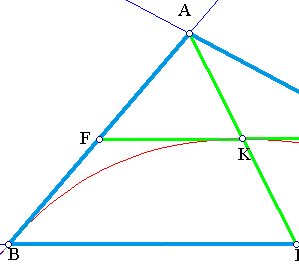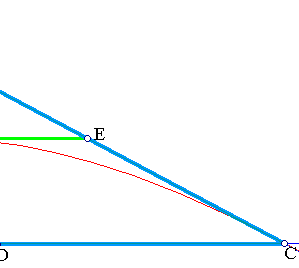##2. Construction in barycentric coordinates

The construction of pBC can be done by means of the bitangent family of conics f(t) = y*z - t*x2.
This describes all conics passing through {B,C} and being there tangent to lines {BA, CA} respectively. The letters represent the lines:
x = 0 (line BC),
y = 0 (line CA),
z = 0 (line AB).
The parabola is precisely the conic-member of this family which passes through point K.
In the standard barycentric coordinate system (x,y,z) represent the coordinates and K has coordinates (1, 1/2, 1/2). This implies that in this system the equation of the parabola is y*z - (1/4)x2 = 0.

##3. Geometric description

The Artzt parabola pBC can be described geometrically also as an envelope of a very easily constructible line.
In fact, consider an arbitrary point D on the basis BC of the triangle and project it parallel to the sides to points C' on AC and B' on AB. The envelope of the diagonal B'C' of the parallelogram DB'AC' is the Artzt parabola pBC.
A proof of this can be found in Artzt_Generation2.html . File Artzt_Generation.html contains a second proof of the same fact using barycentric coordinates.
Another way to generate this parabola can be found in the file ArtztIsosceles.html .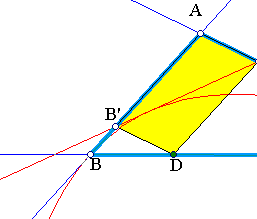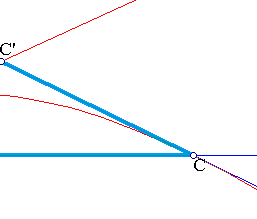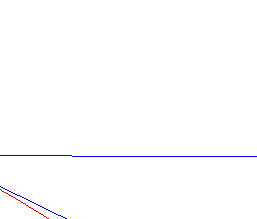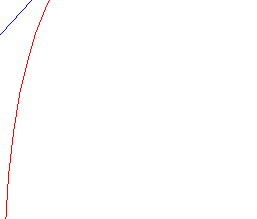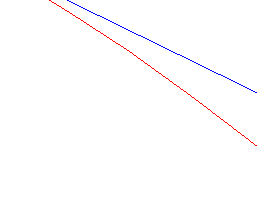Artzt_2.html
Artzt2.html
Artzt_Generation.html
Artzt_Generation2.html
ArtztIsosceles.html
ArtztCanonical.html
ArtztSteiner.html
BrocardSecond.html
IsoscelesIntersection.html
ParabolaChords.html
ParabolaFromProjections.html
ParabolasFromEqualSegs.html